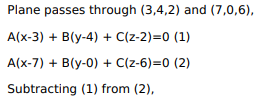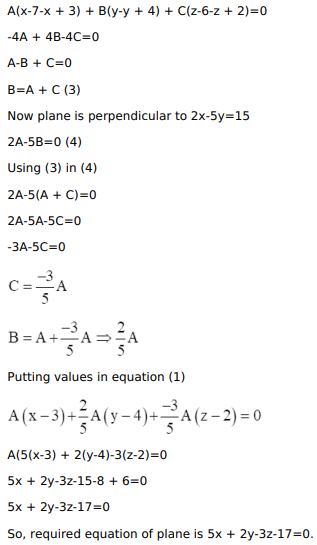# Find the equation of the plane through the pointsQuestion:

Find the equation of the plane through the points $A(3,4,2)$ and $B(7,0,6)$ and perpendicular to the plane $2 x-5 y=15$.

HINT: The given plane is $2 x-5 y+0 z=15$

Solution: